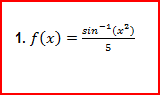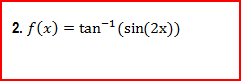Clutch Prep is now a part of Pearson
Ch.7: Derivatives (Part 2)WorksheetSee all chapters

# Derivatives of Inverse Trigonometric Functions

See all sections
Sections
Derivatives Trigonometric Functions
Derivatives of Inverse Trigonometric Functions
Derivatives of Exponential Functions
Derivatives Of Logarithmic Functions
Logarithmic Differentiation

Concept #1: Inverse Trig Derivatives (w/Chain Rule)

###### Expert Q&A

Ask unlimited questions and get expert help right away.

Concept #2: Intro

###### Expert Q&A

Ask unlimited questions and get expert help right away.

Practice: Derive the following function:Practice: Derive the following function:###### Expert Q&A

Ask unlimited questions and get expert help right away.